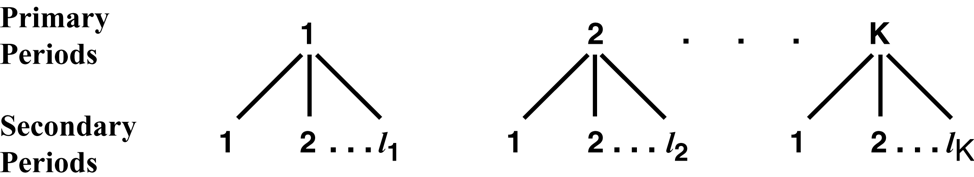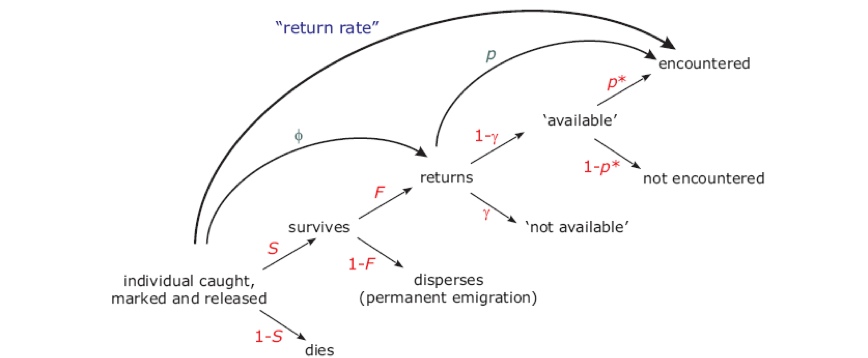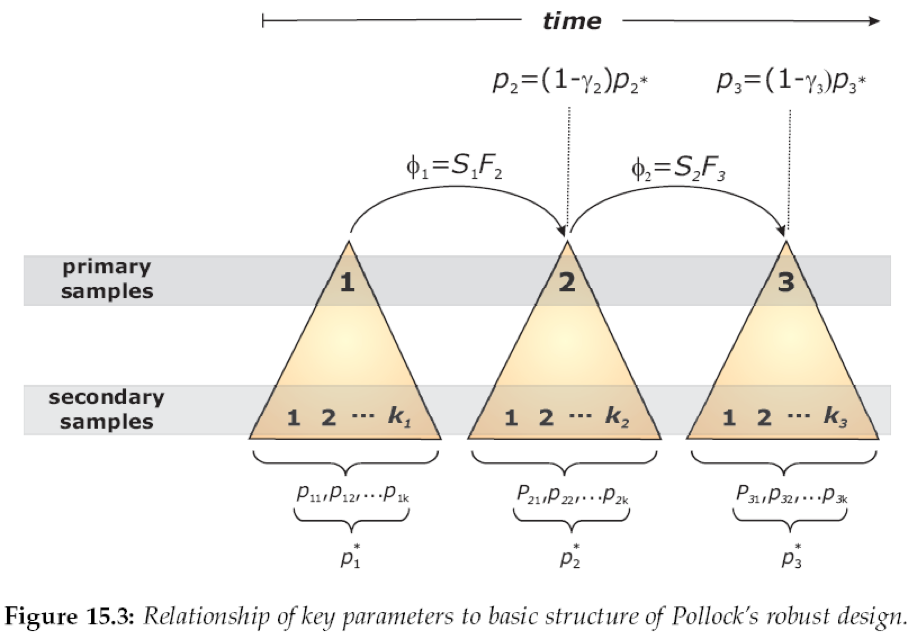Quantities of interest in studies of population dynamics: * State variable – population size * Rate of change in state variable – population growth rate * Vital rates responsible for change in state variable – births, deaths, and movement

To date in this course, we’ve considered models that estimate: * Abundance (closed models) * Could estimate population growth rate if estimate abundance in multiple years, i.e., $$\hat \lambda = \frac{\hat{N_2}}{\hat{N_1}}$$ * Population losses to mortality in CJS and multi-state models * Movement among sampled locations (multi-state models)

With the Robust Design, which was developed by Ken Pollock, you work with sampling at two temporal scales to achieve the following:

• estimate abundance with closed models for data from short-term sampling

• estimate survival from open models using data from longer-term sampling, e.g., annual intervals

• estimate recruitment from the estimates produced for $$\hat{N_t}$$ and $$\hat{S_t}$$

Pollock’s (1982) Robust Design

• $$K$$ primary sampling occasions spaced out such that expect mortality and recruitment between occasions, e.g., 1 year apart

• $$l_i$$ secondary sampling occasions spaced at short intervals within each primary period, $$i$$ (the number of secondary occasions can vary by year)Example of Robust Design:

• $$K = 4$$ or 4 primary periods

• $$l_i = 3$$ for each primary period, i.e., there are 3 secondary occasions within each of the 4 primary occasions

• Example EH for an animal in such a study: 110 001 011 000

• Refer to the EH as \$X_{gij}, which indicates if individual g was captured (1) or not (0) in secondary sampling period $$j$$ of primary period $$i$$.

Use 2 analyses: (1) modeling of live recaptures data (open model, e.g., CJS) and (2) modeling of data within each primary occasion using a closed-population approach (closed model, e.g., $$M(bh)$$). With such an ad hoc approach, you can

• Estimate $$N_i$$ with closed models using data from secondary occasions of primary occasion $$i$$

• Estimate $$\phi_i$$ with open models (uses $$p_i^{open}$$ )

• Estimate the number of new individuals that recruited to the population since the previous primary occasion, $$B_i$$, using estimates of $$N_i$$ and $$\phi_i$$ from previous 2 steps. For example, if $$\hat{N_1} = 100$$, $$\hat{N_2} = 120$$, and $$\hat{\phi_1} = 0.8$$, then $$\hat{B_1} = 40$$: $$120 - (100 \cdot 0.8) = 120 - 80 = 40$$. Note: if you have losses on capture (e.g., individuals die and are not released alive after capture), there are equations for adjusting $$\hat{B_1}$$ accordingly.

• It’s recommended that you use the same structure for your closed model in each of the $$K$$ closed-model data sets as it helps ensure that magnitudes and directions of biases associated with estimates of $$N_i$$ are consistent, which leads to more reasonable estimates of recruitment.

• Even if you need time-varying models for $$\phi$$ and $$p$$, you can now obtain estimates for all occasions because the closed-models can provide a value for $$p$$ on the last occasion.

We will use the ad-hoc approach at the start of the homework because it can help you to gain intuition as to what is happening with the approach. But, using the actual robust-design model is typically a better way to go as all your estimates are contained inside a single analysis.

Two types of $$p$$ in robust design analyses

1. $$p_{i,j}$$ from secondary samples that refer to the probability that an animal is captured on secondary occasion $$j$$ of primary occasion $$i$$ given that it was in the population on that occasion. Closed models allow for lots of flexibility in how you model the $$p_{i,j}$$, e.g., you can model individual heterogeneity.

2. $$p_i^*$$ obtained from primary samples that refer to the probability that an animal is caught at least once in primary occasion $$i$$ (i.e., on at least one of the $$l_i$$ secondary occasions) given that the animal was in the population during that sampling period

• You can also obtain $$p_i^{open}$$ from primary-occasion-only analysis, e.g., CJS

• Can compare what you obtain for $$p_i^*$$ using values of $$p_{i,j}$$ from closed modeling (i.e., secondary occasions within a primary occasion) to what you obtain for $$p_i^{open}$$ from primary-occasion-only analysis (e.g., CJS) to check for evidence of temporary emigration, i.e., with temporary emigration ($$\gamma$$), $$p_i^{open} = (1 - \gamma)\cdot p_i^*$$ (see Figure 15.1 and accompanying figure legend and text in Ch. 15 of CW for a more-detailed explanation).

• For the closed model, $$p_i^* = 1 - \displaystyle \prod_{j = 1}^{l_i}*(1-p_{i,j})$$ - this is the probability that you didn’t miss the individual every time. For the closed model, the $$p_{i,j}$$ are for animals present in the study area.

• For the primary-occasions-only analysis, e.g., CJS, your estimates of $$p_i$$, or what I’m calling here, $$p_i^{open}$$, are alternate estimates of $$p_i^*$$. If you have temporary emigration, then those will be lower than what you obtain working with the $$p_{i,j}$$ from the closed modeling. In reality, with temporary emigration, primary-occasions-only analysis, e.g., CJS, is estimating apparent capture probabilities because the approach can’t distinguish between animals with $$p_i = 0$$ because they are temporary emigrants and animals that were present but not caught. With the ad-hoc version of robust-design modeling, you can compare $$p_i^{open}$$ and $$p_i^*$$ to check for evidence of temporary emigration.

• You can see how it’s possible to learn about temporary emigration, $$\gamma$$, by working with the equation $$p_i^{open} = (1 - \gamma)\cdot p_i^*$$. Rearrange as $$\frac{p_i^{open}}{p_i^*} = (1 - \gamma)$$ and $$\gamma = 1 - \frac{p_i^{open}} {p_i^*}$$. E.g., $$p_i^{open} = 0.4$$ and $$p_i^* = 0.5$$, so $$\gamma = 1 - \frac{0.4}{0.5} = 1 - 0.8 = 0.2$$.

• Now consider how $$p_i^{open} = 0.4$$ results: 20% of the individuals are temporary emigrants and so undetectable, whereas the other 80% are present on the study area with $$p_i^* = 0.5$$ and $$0.8 * 0.5 = 0.4$$.

Full Likelihood Approach to Robust Design

A full likelihood for data from both primary and secondary periods has been developed. That likelihood includes the mathematical relationships among the capture parameters of the likelihood components for the two types of data. Further, there are versions of the likelihood that include temporary emigration, which if it occurs and is ignored introduces bias into estimates. The figure below from Ch 15 of CW, which was written by Bill Kendall, Colorado State University, illustrates the processes underlying an encounter history from a study employing a robust design.From the figure above, you can see that return rate (R) is:

$$R = \phi \cdot p$$, where that version of $$p$$ is $$p_i^{open}$$. Alternatively,

$$R = S \cdot F \cdot [(1-\gamma)\cdot p_i^*]$$, where

• $$S$$: True survival rate

• $$F$$: Fidelity or probability that don’t permanently emigrate from study area

• $$\gamma$$: Probability of temporary emigration from study area

• $$p^*$$: Probability of detection given that the individual is present on the study area

The relationships between the parameters and the robust design’s sampling structure over the primary and secondary occasions are nicely illustrated in Figure 15.3 of CW. Notice that although the robust design allows you to learn about temporary emigration, $$\gamma$$, it does not allow you to disentangle $$S$$ and $$F$$, such that $$\phi$$ remains apparent survival rate. To learn about $$F$$, you would need additional information. For example, you might use information on recoveries of dead animals in harvested population, which could provide information about true survival rate. Or, you might incorporate telemetry data to learn about true survival rate, e.g., use known-fate modeling of survival rate.Some details of the full-likelihood approach

• The likelihood is written as products of components of the 2 types of data (closed & open)

• can include an excellent diversity of open and closed model structures to deal with potential sources of variation in the underlying parameters

• full-likelihood approach allows model-selection to be readily done among competing models of movement process

• if temporary emigration occurs, you can evaluate whether temporary emigration has

• a random pattern – ad-hoc approach also works

• a Markovian pattern (see graphic below) – likelihood approach needed